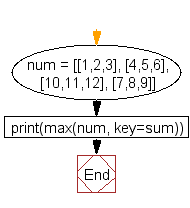﻿ Python: Find the list in a list of lists whose sum of elements is the highest - w3resource# Python: Find the list in a list of lists whose sum of elements is the highest

## Python List: Exercise - 66 with Solution

Write a Python program to find the list in a list of lists whose sum of elements is the highest.

Sample Solution:-

Python Code:

``````num = [[1,2,3], [4,5,6], [10,11,12], [7,8,9]]
print(max(num, key=sum))
```
```

Sample Output:

```[10, 11, 12]
```

Pictorial Presentation:Flowchart:## Visualize Python code execution:

The following tool visualize what the computer is doing step-by-step as it executes the said program:

Python Code Editor:

Have another way to solve this solution? Contribute your code (and comments) through Disqus.

What is the difficulty level of this exercise?

Test your Python skills with w3resource's quiz

﻿

## Python: Tips of the Day

```print(2_000_000)
```2000000